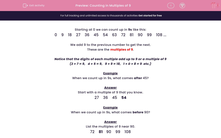# Counting in Multiples of 9

In this worksheet, children must count in multiples of 9 forwards and backwards to find the missing number.This content is premium and exclusive to EdPlace subscribers.Key stage:  KS 2

Curriculum topic:   Maths and Numerical Reasoning

Curriculum subtopic:   Factors and Multiples

Difficulty level:#### Worksheet Overview

Starting at 0 we can count up in 9s like this:

0    9    18    27    36    45    54    63    72    81    90    99    108 ...

We add 9 to the previous number to get the next.

These are the multiples of 9.

Notice that the digits of each multiple add up to 9 or a multiple of 9

(2 + 7 = 9,   4 + 5 = 9,   9 + 9 = 18,   1 + 0 + 8 = 9  etc.)

Example

When we count up in 9s, what comes after 45?

27    36    45    54

Example

When we count up in 9s, what comes before 90?